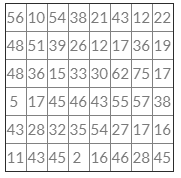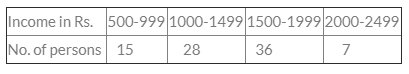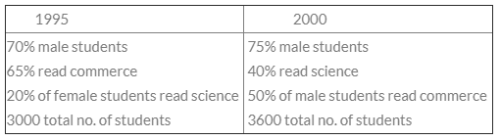# Test: Statistical Description Of Data - 4

## 40 Questions MCQ Test Quantitative Aptitude for CA CPT | Test: Statistical Description Of Data - 4

Description
Attempt Test: Statistical Description Of Data - 4 | 40 questions in 40 minutes | Mock test for CA Foundation preparation | Free important questions MCQ to study Quantitative Aptitude for CA CPT for CA Foundation Exam | Download free PDF with solutions
QUESTION: 1

Solution:
QUESTION: 2

Solution:
QUESTION: 3

### In Histogram if the classes of unequal width then the heights of the rectangles must be proportional to the frequency densities.

Solution:
QUESTION: 4

In representing simple frequency distributions of a discrete variable

Solution:
QUESTION: 5

Class boundaries should be considered to be the real limits for the class interval

Solution:
QUESTION: 6

“Cumulative Frequency” only refers to the

Solution:
QUESTION: 7

Most extreme values which would ever be included in a class interval are called

Solution:
QUESTION: 8

The number of types of cumulative frequency is

Solution:
QUESTION: 9

Frequency density is used in the construction of

Solution:
QUESTION: 10

Vertical bar chart may appear somewhat alike

Solution:
QUESTION: 11

In the construction of a frequency distribution, it is generally preferable to have classes of

Solution:
QUESTION: 12

For determining the class frequencies it is necessary that these classes are

Solution:
QUESTION: 13

Frequency density is used in the construction of

Solution:
QUESTION: 14

The number of observation falling within a class is called

Solution:
QUESTION: 15

For the construction of a grouped frequency distribution

Solution:
QUESTION: 16

In all Statistical and diagrams involving end points of classes

Solution:
QUESTION: 17

Tally marks determines

Solution:
QUESTION: 18

The number of errors in Statistics are

Solution:
QUESTION: 19

The number of “ Frequency distribution” is

Solution:
QUESTION: 20

The graphical representation of a cumulative frequency distribution is called

Solution:
QUESTION: 21

When all classes have a common width

Solution:
QUESTION: 22

An area diagram is

Solution:
QUESTION: 23

(Class frequency) / (width of the class) is defined as

Solution:
QUESTION: 24

An approximate idea of the shape of frequency curve is given by

Solution:
QUESTION: 25

Cumulative Frequency Distribution is a

Solution:
QUESTION: 26

A table has

Solution:
QUESTION: 27

In general the number of types of tabulation are

Solution:

There are two types of tabulation methods :

a)simple tabulation

b)complex tabulation.

QUESTION: 28

Unequal widths of classes in the frequency distribution do not cause any difficulty in the construction of

Solution:
QUESTION: 29

To find the number of observations less than any given value

Solution:
QUESTION: 30

Ogive is a

Solution:
QUESTION: 31

The most common form of diagrammatic representation of a grouped frequency distribution is

Solution:
QUESTION: 32

The following data relate to the marks of 48 students in statistics:What are the frequency densities for the class interval 30-39, 40-49 and 50-59

Solution:
QUESTION: 33

The relationship between two variables are shown in

Solution:
QUESTION: 34

The following data relate to the incomes of 86 persons:What is the percentage of persons earning more than Rs. 1500?

Solution:
QUESTION: 35

Cost of sugar in a month under the heads Raw Materials, labour, direct production and others were 12, 20, 35 and 23 units respectively. What is the difference between the central angles for the larges and smallest components of the cost of sugar?

Solution:
QUESTION: 36

In a study about the male and female students of commerce and science departments of a college in 5 years, the following dates were obtained:After combining 1995 and 2000 if x denotes the ratio of female commerce students to female Science student and y denotes the ratio of male commerce student to male Science student, then

Solution:
QUESTION: 37

Out of 1000 persons, 25 per cent were industrial workers and the rest were agricultural workers. 300 persons enjoyed world cup matches on TV. 30 per cent of the people who had not watched world cup matches were industrial workers. What is the number of agricultural workers who had enjoyed world cup matches on TV?

Solution:
QUESTION: 38

Details are shown by

Solution:
QUESTION: 39

Graph is a

Solution:
QUESTION: 40

A sample study of the people of an area revealed that total number of women were 40% and the percentage of coffee drinkers were 45 as a whole and the percentage of male coffee drinkers was 20. What was the percentage of female not-coffee drinkers?

Solution:Use Code STAYHOME200 and get INR 200 additional OFF Use Coupon Code